Processing ......FreeComputerBooks.com Links to Free Computer, Mathematics, Technical Books all over the World

Matters Computational: Ideas, Algorithms, Source Code
Top Free Data Science Books 🌠 - 100% Free or Open Source!
• Title: Matters Computational: Ideas, Algorithms, Source code
• Author(s) Jorg Arndt
• Publisher: Springer; 1st Edition (December 23, 2010); eBook (Creative Commons Edition)
• Hardcover: 978 pages
• eBook: PDF, ePub, Kindle, PostScript, DVI, etc.
• Language: English
• ISBN-10/ASIN: 3642147631
• ISBN-13: 978-3642147630Book Description

This book provides algorithms and ideas for computationalists, whether a working programmer or anyone interested in methods of computation. The focus is on material that does not usually appear in textbooks on algorithms.

Subjects treated include low-level algorithms, bit wizardry, combinatorial generation, fast transforms like the Fourier transform, and fast arithmetic for both real numbers and finite fields. Various optimization techniques are described and the actual performance of many given implementations is examined. The focus is on material that does not usually appear in textbooks on algorithms. The implementations are done in C++ and the GP language, written for POSIX-compliant platforms such as the Linux and BSD operating systems.

Where necessary the underlying ideas are explained and the algorithms are given formally. It is assumed that the reader is able to understand the given source code, it is considered part of the text. We use the C++ programming language for low-level algorithms. However, only a minimal set of features beyond plain C is used, most importantly classes and templates. For material where technicalities in the C++ code would obscure the underlying ideas we use either pseudocode or, with arithmetical algorithms, the GP language. Appendix C gives an introduction to GP.

Example computations are often given with an algorithm, these are usually made with the demo programs referred to. Most of the listings and fgures in this book were created with these programs. A recurring topic is practical efficiency of the implementations. Various optimization techniques are described and the actual performance of many given implementations is indicated.

• Jorg Arndt: born 1964 in Berlin, Germany. Study of theoretical physics at the University of Bayreuth, and the Technical University of Berlin, Diploma in 1995. PhD in Mathematics, supervised by Richard Brent, at the Australian National University, Canberra, in 2010.
Reviews, Ratings, and Recommendations: Related Book Categories: Read and Download Links:Similar Books:
•Models of Computation: Exploring the Power of Computing

It covers the traditional topics of formal languages, automata and complexity classes, as well as an introduction to the more modern topics of space-time tradeoffs, memory hierarchies, parallel computation, the VLSI model, and circuit complexity.

•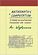Mathematics and Computation (Avi Wigderson)

This book provides a broad, conceptual overview of computational complexity theory - the mathematical study of efficient computation. With important practical applications to computer science and industry, computational complexity theory, etc.

•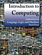Introduction to Computing: Language, Logic, and Machines

This book focuses on how to describe information processes by defining procedures, how to analyze the costs required to carry out a procedure, and the fundamental limits of what can and cannot be computed mechanically.

•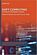Soft Computing: Techniques in Engineering Sciences

Soft Computing is used where a complex problem is not adequately specified for the use of conventional math and computer techniques. This book elaborates on the most recent applications of Soft Computing in various fields of engineering.

•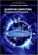Quantum Computing: Progress and Prospects

Provides an introduction to the Quantum Computing, including the unique characteristics and constraints of the technology, and assesses the feasibility and implications of creating a functional quantum computer capable of addressing real-world problems.

•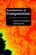Foundations of Computation (Carol Critchlow, et al)

It includes topics from discrete mathematics, automata theory, formal language theory, and the theory of computation, along with practical applications to computer science. The course has no prerequisites other than introductory computer programming.

•Introduction to Theory of Computation (Anil Maheshwari, et al)

This is a textbook on formal languages, automata theory, and computational complexity. This text strikes a good balance between rigor and an intuitive approach to computer theory.

•Computing Fundamentals: Theory and Practice of Software Design

The book introduces the reader to computer programming, i.e. algorithms and data structures. It covers many new programming concepts that have emerged in recent years including object-oriented programming and design patterns.

•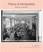Theory of Computation, Making Connections (Jim Hefferon)

The book is geared toward those who thirst for computation theory knowledge. To cater to the demands of a wide range of people, the principles in this book are explained in a way that is easy to understand, digest and apply in the upcoming career.

•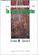Introduction to Theory of Computation (Eitan M. Gurari)

This book explores some of the more important terminologies and questions concerning programs, computers, problems, and computation. The exploration reduces in many cases to a study of mathematical theories, such as those of automata and formal languages; theories that are interesting also in their own right.

Book Categories
 :All CategoriesTop Free BooksRecent BooksMiscellaneous BooksComputer EngineeringComputer LanguagesComputer ScienceData Science/DatabasesJava and Java EE (J2EE)Linux and UnixMathematicsMicrosoft and .NETMobile ComputingNetworking and CommunicationsSoftware EngineeringSpecial TopicsWeb Programming
Other Categories